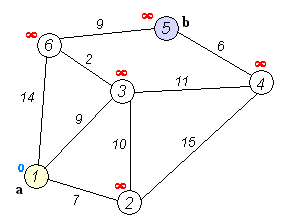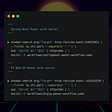# Pascal RSA Writeup. K3RN3L CTF

## Foreword

K3RN3L CTF was really cool this year, there were a lot of fascinating crypto challenges. Too bad, I was busy this weekend, so I only took a brief view on a couple of them and solved just one, which is the challenge I’m going to explain.

## Challenge description

So we are given the source code and the data:

Let’s break down the code. First, it generates some 20-bit prime `p` and generates Pascal’s triangle until its last row’s length reaches `p` (so it generates `p` rows). After that, this last row of triangle is being converted to binary string of residues modulo 2 of each number in the row. This binary string is used to create number `d`.

After all that we see pretty standard RSA initialization. Code generates `P` and `Q` until GCD of `(P — 1) * (Q — 1)` and `d` equals to 1 (so `d` has an inverse modulo Euler’s function of N). Once it is done the modulo and public exponent are calculated and flag is encrypted with public exponent and modulo.

Additional data to challenge is `p`, encrypted flag and modulo.

## Solution

So to decrypt the flag all we need to find is `d`. And we are actually already given the code to calculate it. We could just copy it and run. But wait a minute… Notice how the calculation is done. It calculates the whole Pascal triangle with `p` rows. Our `p` is `751921`, therefore the code will create `751921` arrays with sizes `1, 2, 3, ..., 751921`. That’s a lot of memory (and a lot of computations, although memory for now is much more crucial), my laptop has only 12GB of RAM, so I it doesn’t have enough memory to run this code.

Next thing I thought was: “All right, if we can’t calculate the whole triangle, let’s calculate just one row as that’s all we need”. A bit of googling yields us the following code:

This code is pretty efficient, it calculates just one array, instead of hundreds of thousands. Still, when I run this code, my laptop got freezed, because the code has quickly ate all of the RAM. Seems like we have to try something else.

Now let’s consider this. We don’t need to calculate the row itself, we need to calculate just the number it represents as a binary string of residues modulo 2. Well, let’s then calculate just one number. We will use the same code, but instead of creating an array, let’s create the number itself:

Now the code doesn’t require additional memory. The downside is that it requires a lot of time to calculate the result. It takes somewhat about 10 minutes on my laptop to calculate `d`. We could live with that and the code to decrypt the flag would look like this:

But, let’s try to speed it up. Now let’s reconsider our task again. Do we have to calculate the row of Pascal’s triangle itself? No, we need to calculate the number it represents as a residues modulo 2. But we spend time to calculate each number in the row, and these numbers grow really fast, so the calculation becomes slow with large rows. But what if we could just calculate the residues? Is it possible? Turns out, it is. A bit of googling gives us the Sierpinski’s triangle, apparently that’s exactly what we need. Now we have the formula to find the exact number the Pascal’s triangle row represents as a binary strings of residues modulo 2:

This might look a bit scary and expensive to calculate, but actually it works really fast. Notice that we need to calculate powers 3 times. But two times we calculate the powers of two: `2**i` and `2**(2 ** i)` . Calculation of power of `2` could be done highly efficient just shifting bits to the left. So `2**i` might be calculates as `1 << i`, and `2**(2 ** i)` as `1 << (1 << i)`. And one other power is either `1` or `0`, because of modulo 2. Without powers, there are just log(N) additions and log(N) multiplications. So our optimized code now looks as follows:

And it takes only 2.5 seconds to find the flag now:

--

--

--

## More from 0awawa0

awawa.fun

Love podcasts or audiobooks? Learn on the go with our new app.

## Step into Parallel computing## How I TRIED to Play With Flappy Bird With My BRAIN (And Failed Miserably)## Building Autonomous Drone in Python Part 1## Dijkstra’s algorithm## Weaponizes nuclei Workflows to Pwn All the Things## Don’ts in JDBC Primary user storeawawa.fun

## Hackthebox Horizontall machine writeup## TryHackMe Writeup : Solar, exploiting Log4J🥷🏻🥷🏻## TryHackMe walkthrough — Hydra## “Tricking” our developers into liking application security